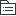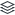# 客服部工作安排

48
|
4

## 相关模板推荐

•## 客服部 —— 作品大纲

•平台
• 京东
• 天猫
• C店
• 唯品会
• 考拉
•职能
• 售前
• 售中
• 售后
•品牌
• 达衣岩
• 旗舰店
• C店
• 京东
• 唯品会
• 目前为入仓
• 考拉
• CRZ
• 旗舰店
• 京东
• 分销
• 唯品会
•班次
• 行政班
• 主管级
• 彬彬
• 佳婷
• 早班
• 售前客服
• 售后客服
• 中班
• 售前客服
• 售后客服
• 晚班
• 售前客服
• 售后待定
• 待新人成长一些再定（双十二之后）
• 加班
• 日常加班
• 活动加班
• 休息
• 正常休息
• 调休
•分工
• 管理
• 佳婷
• 彬彬
• 老员工
• 小胡
• 珍珍
• 建明
• 小敏
• 新员工
• 子龙
• 小乔
• 彭洁
• 小建明
• 小坚
• 杨杰
• 小权
•奖惩
• 绩效加分项
• 绩效减分项
• 扣罚
•流程
• 时间项
• 账户分流（售前客服）
• 工作日/休息日
• 对应班表的分流同事负责分流给当班客服
• 10：30主动检查（售前客服主管）
• 分流好后当班客服主动向上反馈完成
• 上午请假
• 请假前先确定交接给哪位同事来做（请假人）
• 10：30主动检查（售前客服主管）
• 分流好后当班客服主动向上反馈完成
• 批复请假单前，确定交接人可以操作（售前主管）
• 10：30主动检查（售前客服主管）
• 分流好后当班客服主动向上反馈完成
• 每日报表、截图（售前主管）
• 2个天猫店制作
• 2个京东店截图
• 发送
• 发送客服群
• 数据异常、单项排名最末的客服主动通知管理
• 响应时间最末
• 告知加强
• 加音箱（考虑均值偏离情况、个人进步情况、问题出现频次）
• 区分售前、售后
• 绩效参考项
• 回复率最末
• 告知加强并问清原因
• 绩效参考项
• 转化率项后续调整确定
• 发送给小马哥
• 聊天记录检查（售前、售后）
• 目前忙，空下来执行
• 跟进发货、缺货（售前）
• 发货（售前跟进）
• 昨日订单是否有发出（日常）
• 已发出部分
• 完结
• 未发出部分可否发出
• 可发出
• 催发
• 昨日审单部分
• 上午11：00催发一次
• 下午15：00或16：00再次查看结果
• 已发出
• 完结
• 仍未发出
• 电话联系
• 不可发出
• CRZ
• 调货部分
• 有调到货
• 解锁库存发货
• 发出
• 完结
• 被其他平台占用
• 继续调货
• 隔天再看
• 没调到货
• 无货
• 转缺货处理
• 自己仓部分
• 达衣岩
• 无货
• 转缺货处理
• 历史遗留订单是否可发出
• 可发出
• 发出并完结
• 不可发出
• 周末发货情况
• CRZ
• 确定周六日对方休息情况
• 达衣岩
• 周五下午催下当天16：00前订单一定要发完
• 周六周日优先京东、考拉、唯品
• 日常周六日一个售前11：00、下午15：00排查一次
• 如15：00排查仓库已发出
• 完结
• 15：00排查依旧未发出
• 再次催
• 私聊
• 电话联系
• 活动期
• 提前2天告知对方需加班
• 缺货
• 系统有货
• 联系仓库找货
• 找到了
• 加急当天发
• 找不到
• 要求仓库做库存差异
• 转移库存至差异仓（仓库操作）
• 分平台处理
• 天猫
• 联系顾客劝改色改码
• 同意
• 在系统里给客户修改
• 发出
• 完结
• 跟黄磊和CRZ的IT确定，如果同步在平台修改，是否会造成系统发货异常
• 不会
• 在平台里也同步修改
• 修改流程
• 不同意
• 做退款流程
• 同意退款
• 引导退款
• 退款
• 完结
• 不同意退款（等）
• 等吧
• C店
• 联系顾客劝改色改码
• 同意
• 在系统里给客户修改
• 发出
• 完结
• 跟黄磊和CRZ的IT确定，如果同步在平台修改，是否会造成系统发货异常
• 不会
• 在平台里也同步修改
• 修改流程
• 不同意
• 做退款流程
• 同意退款
• 引导退款（退款原因引导“不想要了/不喜欢”）（一笔带过，给客服做讲解培训）（统一话术并严格要求按话术发送）
• 退款
• 完结
• 不同意退款（等）
• 等吧
• 客户投诉
• 电话联系
• 能联系上
• 赠品优惠券引导
• 客户同意
• 联系客户撤销投诉
• 系统做单，赠品发出
• 客户不同意
• 向上级主管说明情况并确定是否可以现金补偿，以及补偿金额
• 再次联系客户
• 按约定的金额进行现金补偿引导
• 同意
• 客户撤诉
• 打款
• 不同意
• 反馈上级领导报备
• 联系不上
• 短信留言
• 旺旺联系
• 赠品优惠券引导
• 客户同意
• 联系客户撤销投诉
• 系统做单，赠品发出
• 客户不同意
• 向上级主管说明情况并确定是否可以现金补偿，以及补偿金额
• 再次联系客户
• 按约定的金额进行现金补偿引导
• 同意
• 客户撤诉
• 打款
• 不同意
• 反馈上级领导报备
• 京东
• 联系顾客劝改色改码
• 同意
• 在系统里给客户修改
• 发出
• 完结
• 跟黄磊和CRZ的IT确定，如果同步在平台修改，是否会造成系统发货异常
• 不会
• 在平台里也同步修改
• 修改流程
• 不同意
• 做退款流程
• 同意退款
• 引导退款（退款原因引导“不想要了/不喜欢”）（一笔带过，给客服做讲解培训）（统一话术并严格要求按话术发送）
• 退款
• 完结
• 不同意退款（等）
• 向上级主管报备情况
• 按实际情况灵活处理（客服主管）
• 客户投诉
• 电话联系
• 能联系上
• 赠品优惠券引导
• 客户同意
• 联系客户撤销投诉
• 系统做单，赠品发出
• 客户不同意
• 向上级主管说明情况并确定是否可以现金补偿，以及补偿金额
• 再次联系客户
• 按约定的金额进行现金补偿引导
• 同意
• 客户撤诉
• 打款
• 不同意
• 反馈上级领导报备
• 联系不上
• 短信留言
• 咚咚联系
• 赠品优惠券引导
• 客户同意
• 联系客户撤销投诉
• 系统做单，赠品发出
• 客户不同意
• 向上级主管说明情况并确定是否可以现金补偿，以及补偿金额
• 再次联系客户
• 按约定的金额进行现金补偿引导
• 同意
• 客户撤诉
• 打款
• 不同意
• 反馈上级领导报备
• 注意：联系的过程中千万不要提及“亲，淘宝天猫唯品会，支付宝”等京东以外平台的名称以及信息
• 唯品会
• 联系运营、商品
• 催运营、商品确定是否报备
• 需报备
• 客服操作报备
• 告知小马哥
• 考拉
• 联系运营、商品
• 暂时完结
• 系统没货
• 联系商品看是否其他仓可以调货
• 调不到货
• 能调到货
• 联系仓库加急发出
• 核对异常件（售后）
• 群内收货异常
• 少件
• 电话联系客户核实是否少件
• 让顾客线上旺旺第一时间联系客服，保留承认少件聊天记录截图证据
• 群内通知仓库签收
• 后台退款流程
• 收到货的子订单正常退款
• 未收到货的子订单
• 拒绝退款
• 让客户补货款
• 已补款
• 退款
• 未补款或不补款
• 拒绝退款
• 客户撤销退款
• 初期联系上级主管报备
• 测试闺蜜投诉
• 不是
• 群内通知仓库拒收包裹
• 可拒收
• 拒绝退款
• 旺旺留言并告知客户拒收原因
• 不可拒收（流程不合理，后续需要优化）
• 快递开证明
• 可开
• 不可开
• 报备给上级领导
• 退款给客户
• 表格记录并一定要在原订单上做备注
• 告知小马哥
• 称重
• 报备上级主管
• 测试云标签打标
• 颜色尺码不符
• 通知仓库查看衣服是否破损、是否影响二次销售
• 有破损，影响二次销售
• 看备注是否已跟客户达成一致退货
• 之前备注及聊天内容未达成一致
• 通知仓库拒收
• 查看是否买家申请退换货
• 无申请的
• 通过唯一码、电话、收件人姓名确定是否可以确定顾客
• 能确定
• 旺旺联系客户告知已拒收此包裹
• 不能确定
• 客服登记无头件，等客户来找（待细化）
• 有申请的
• 拒绝退款、上传破损影响二次销售的凭证
• 之前备注及聊天内容已达成一致
• 在系统里修改退货单
• 通知仓库签收
• 要求仓库入库并转次品仓
• 给客户进行退换
• 无破损、不影响二次销售
• 在系统里修改退货单
• 通知仓库签收
• 给客户进行退换
• 破损、污渍
• 到付件
• 确认顾客
• 能确认客户
• 查看备注以及聊天记录看是否与客户达成一致可发到付件
• 与客户达成一致、可发到付件退回
• 通知仓库签收
• 顺丰月结快递（无需仓库垫款）
• 其他快递（需仓库垫付快递费
• 登记打款表格（登记顾客信息，并打款给仓库垫付运费的同事）
• 未与客户达成可发到付件协议
• 非商家责任的退换货
• 联系客户是否愿意补款
• 愿意
• 线上补款
• 通知仓库签收
• 不愿意
• 判断客户属性
• 优质客户（通知仓库签收）
• 大金额订单退单件客户
• 多件产品退单件客户
• 高购买频次老客户
• 普通客户（通知仓库拒收）
• 单件客户
• 初次购买低金额
• 全买全退客户
• 劣质客户
• 商家责任的退换货
• 不能确认客户
• 通知仓库拒收
• 客服登记无头件（待细化）
• 非公司产品
• 少吊牌
• 款式不符
• 表格反馈异常
• 18点前等仓库回传
• 未回传
• 电话联系仓库负责人并确定是否需要等待
• 已回传
• 21点前处理
• 处理完毕部分
• 21点前上传群内并告知仓库
• 无法处理部分
• 历史遗留异常
• 处理各种退货退款、仅退款（售后）
• 截图群里@主管
• 核对问题售后单、联系客户（售后）
• 后台盘查（售前、售后）
• 售前
• 对应人员的回复率、响应时间是否正常
• 有异常
• 无异常
• 完结
• 对应人员近期的成功率是否正常
• 未发货订单是否有处理
• 售前指标数据类
• 售后
• 对应人员的回复率、响应时间是否正常
• 后台是否出现介入、违规、投诉
• 后台是否有快要超时的介入、退款、违规、投诉
• 换货东单的情况
• 售后指标数据类
• 晓多使用情况
• 是否正常开启、使用
• 开启是否正常
• 是否正常启用选项
• 催单是否正常
• 是否有接到异常机器人回复的反馈
• 没有收到
• 盘查聊天记录时查看是否有异常
• 有异常
• 转修改项
• 截图发给对应当事人并告知下次必须反馈此事
• 无异常
• 完结
• 有收到
• 确定是否可以修改
• 可以
• 修改并截图发到群里说明情况
• 不确定
• 咨询小马哥是否可以修改
• 不可以
• 改成非自动回复方式
• 通知对应客服
• O2O情况发送
• 截图、数据统计发微信群
• 无异常
• 完结
• 有异常
• 私聊凤仙
• 处理换货订单（售后）
• 打款以及优惠券发放（售后）
• 周例会
• 时间
• 参会人数
• 内容
• 请款与报销
• 每周五
• 发票开具
• 新流程
• 下月排班（月末）
• 新老客服是否安排合理
• 休息时间分布是否安排合理
• 各个店铺旺旺、咚咚分工明确注明
• 售后分工是否安排合理
• 休息日分流由谁来执行
• 对账（月末）
• 月度总结（月末或下月初）
• 客服绩效
• 发票开具
• 老流程
• 新流程
• 丢件统计
• 客服培训
• 先满足一次
• 争取每月两次
• 活动项
• 预热
• 话术更变
• 利益点确定
• 值班安排
• 正式
• 利益点、催单执行到位
• 分流合理、确保售前销售最大化
• 日常（活动收尾）
• 话术更换回日常（售前）
• 及时处理退货
• 登记发放赠品
• 拍下运费连接
• 备注
• 发出
• 跟进异常退货
• 跟进异常发货
• 人员项
• 现有人员管理
• 分工调整
• 专项提升
• 日常规范
• 新人入职管理
• 规则
• 天猫、淘宝规则
• 京东、唯品规则
• 流程
• 聊天工具
• 系统
• 工作技巧
• 离职员工收尾
• 子账号停用
• 分流停止
• 排班顶替
• 后备人员工作顶替
• 订单项
• 售前
• 咨询
• 未成交
• 下单
• 晓多自动催单
• 客户无回复
• 完结
• 客户有回复
• 继续解决问题
• 客户直接下单
• 有特殊需求
• 备注
• 细则、姓名、时间
• 无特殊需求
• 发货
• 售中
• 已付款状态
• 正常订单
• 发货（时间）
• 非正常订单
• 无货
• 延迟发货
• 缺货
• 已发货揽收物流记录
• 联系仓库调视频
• 无视频
• 仓库问题
• 有视频
• 圆通问题
• 联系圆通核实
• 赔付、登记
• 已发货有物流记录
• 催派送
• 联系快递催促
• 回复客户
• 卡住多日
• 联系快递
• 未解决
• 隔日联系快递
• 回复客户
• 已解决
• 回复客户
• 回复客户
• 售后
• 发票补发
• 已付款状态
• 退款
• 达衣岩AG自动处理
• 下班前整体检查
• 处理成功
• 完结
• 未处理成功
• 反馈客服主管
• 客服主管与IT沟通
• IT提供解决方案
• 其他非AG类店铺
• 下班前整体检查
• 手动退款频次
• 已发货状态
• 仅退款
• 有揽收记录
• 无揽收记录
• 退货退款
• 交易成功状态
• 仅退款
• 部分退款
• 退全款
• 与客户核实
• 与主管核实
• 退货退款
• 换货
• 介入
• 投诉
• 违规
•衔接
• 客户
• 售前客户
• 售后类
• 纠纷类
• 非正常客户
• 对外
• 品牌仓
• 发货问题
• 调货问题
• 可能双十一之后达衣岩退货也要沟通对方
• 核实款式细节
• O2O
• 发货问题
• 收退货问题
• 催促类问题
• 异常类
• 丢件赔偿给谁？
• 快递费用结算解答
• 平台
• 投诉类
• 违规类
• 纠纷类
• 快递
• 合作快递（圆通、顺丰）
• 截停返
• 催发送货
• 理赔
• 快递异常、已签收实际未签收
• 非合作快递（德邦）
• 退回包裹
• 催派送
• 快递异常、已签收实际未签收
• 退货快递（中通、天天、韵达等）
• 正常签收
• 拒收
• 提供证明
• 拒收后再派送
• 到付
• 工商局（工商投诉）
• 举证
• 描述不符需要跟买家写上
• 广告法则直接做书面说明
• 品牌方
• 每月发票
• IT
• 品牌方IT
• 库存问题
• 无权限需要查询表格
• 待补充场景
• 唯一码查询
• 权限问题
• 新增账号问题
• 开发商IT
• CRZ上海
• 逻辑问题
• BUG问题
• 达衣岩黄磊
• 逻辑问题
• BUG问题
• 对内
• 乐资仓
• 发货问题
• 收退件问题
• 调货问题
• 退货仓转正常仓
• 库存问题（准确性）
• 找件？
• 核实款式细节
• 询问物料准备
• 商品
• 页面问题
• O2O、品牌仓沟通反馈
• 款式异常
• 库存问题
• 准确性
• 各仓同步比例
• 安全库存
• 运营
• 活动相关
• 利益点
• 节奏
• 月节奏表
• 单次活动加班需求
• 开场语
• 赠品
• 平台规则相关
• 数据异常相关
• 平台对接相关
• IT
• 系统问题
• 系统需求
• 功能新增
• 硬件问题
• 电脑
• 配件
• 系统
• 网络
• 财务
• 对账
• 已完结订单
• 客服部门可自行处理
• 需要其他环节协助
• 仓库
• 乐资仓库
• 品牌仓
• O2O门店
• 平台小二
• 快递
• 发货的圆通
• 退件的其他快递
• 未完结订单
• 约定时间，订单完结后再处理
• 报销
• 备用金请款
• 日常报销
• 打车等